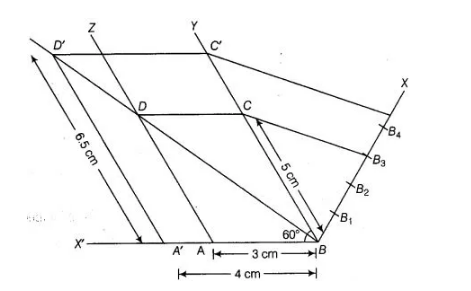# Draw a parallelogram ABCD in which BC = 5 cm,

Question:

Draw a parallelogram $A B C D$ in which $B C=5 \mathrm{~cm}, A B=3 \mathrm{~cm}$ and $\angle A B C=60^{\circ}$, divide it into triangles $B C D$

and ABD by the diagonal BD. Construct the triangles BD'C' similar to $\triangle B D C$ with Scale factor $\frac{1}{4}$. Draw the line segment D'A' parallel

to DA, where A' lies on extended side BA. IS A'BC'D' a parallelogram?

Solution:

Steps of construction

1. Draw a line segment AB = 3 cm.
2. Now, draw a ray BY making an acute ∠ABY = 60°.
3. With B as centre and radius equal to 5 cm draw an arc cut the point C on
4. Again draw a ray AZ making an acute ∠ZAX’ = 60°.                [∴ BY || AZ, ∴ ∠YBX’ = TAX’ = 60°]
5. With A as centre and radius equal to 5 cm draw an arc cut the point D on AZ.6. Now, join $C D$ and finally make a parallelogram $A B C D$

7. Join BD, which is a diagonal of parallelogram ABCD

8. From B draw any ray BX downwards making an acute $\angle \mathrm{CBX}$.

9. Locate 4 points $B_{1}, B_{2}, B_{3}, B_{4}$ on $B X$, such that $B B_{1}=B_{1} B_{2}=B_{2} B_{3}=B_{3} B_{4}$,

10. Join $B_{4} C$ and from $B_{3} C$ draw a line $B_{4} C^{\prime} \| B_{3} C$ intersecting the extended line segment $B C$ at $C^{\prime}$.

11. From point $C^{\prime}$ draw $C^{\prime} D^{\prime} \| C D$ intersecting the extended line segment $B D$ at $D^{\prime}$. Then, $A D^{\prime} B C^{\prime}$ is the required triangle whose sides are $\frac{4}{3}$ of the corresponding sides of $\triangle D B C$

12. Now draw a line segment D'A' parallel to DA, where A' lies on extended side BA i.e ray BX'.

13. Finally, we observe that $A^{\prime} B C D^{\prime}$ is a parallelogram in which $A^{\prime} D^{\prime}=6.5, \mathrm{~cm} A^{\prime} B=4 \mathrm{~cm}$ and $\angle A^{\prime} B D^{\prime}=60^{\circ}$ divide it into triangles BCD' and $A^{\prime} B D^{\prime}$ by the diagonal $B D$.